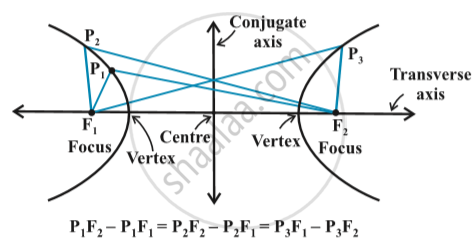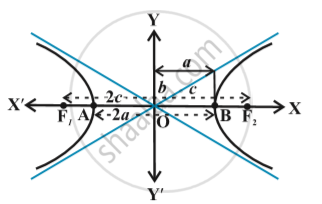# Hyperbola

## Definition

A hyperbola is the set of all points in a plane, the difference of whose distances from two fixed points in the plane is a constant.

## NotesThe term “difference” that is used in the definition means the distance to the farther point  minus the distance to the closer point. The two fixed points are called the foci of the hyperbola. The mid-point of the line segment joining the foci is called the centre of the hyperbola. The line through the foci is called the transverse axis and the line through the centre and perpendicular to the transverse axis is called the conjugate axis. The points at which the hyperbola intersects the transverse axis are called the vertices of the hyperbola in the above fig.The distance between the two foci by 2c, the distance between two vertices (the length of the transverse axis) by 2a and we define the quantity b as b = sqrt(c^2-a^2)
Also 2b is the length of the conjugate axis in above fig.
To find the constant P_1F_2 – P_1F_1 :

By taking the point P at A and  B   in the  above Fig,  we have
BF_1  – BF_2 =  AF_2 – AF_1
(by the definition of the hyperbola)
BA +AF_1– BF_2 = AB + BF_2– AF_1
i.e., AF_1 =  BF_2
So that,  BF_1 – BF_2 =  BA + AF_1– BF_2 = BA = 2a

If you would like to contribute notes or other learning material, please submit them using the button below.